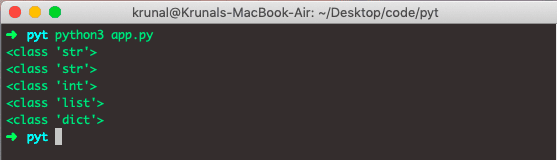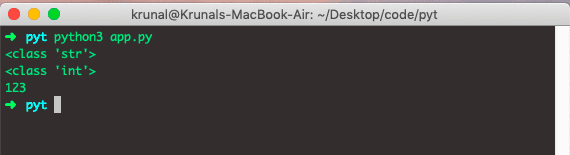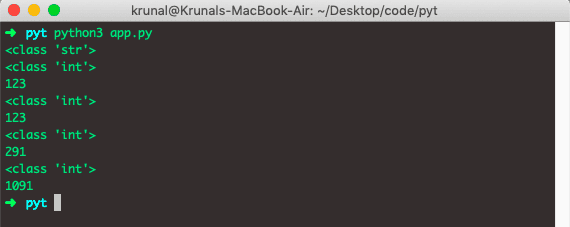# How to Convert String to Integer in Python

Integers in Python are whole numbers. In other words, they have no fractional component. Python offers two data types to store an integer.

1. int
2. string

## How to represent an integer in String in Python

To represent an integer using a string literal in Python, you can put the integer inside the single-quote or double-quotes.

```v1 = "1"
v2 = "11"

print('The value of v1 is: ', v1)
print('The data type of v1 is:', type(v1))
print('The value of v2 is: ', v2)
print('The value of v2 is: ', type(v2))
```

#### Output

```python3 app.py
The value of v1 is:  1
The data type of v1 is: <class 'str'>
The value of v2 is:  11
The value of v2 is:  <class 'str'>```

Python interpreter understands that you want to store the integers 1 and 11 as strings. So you call it with the String containing the number as the argument, and it returns the number converted to an integer.

## Python data type conversion

Data type conversion, also known as type casting, converts a value from one data type to another.

Python provides built-in methods to convert a value from one data type to another. Converting one data type to another is called type casting or conversion.

Python needs to store different sorts of data using different data types. Several data types in Python are used to store data, including numbers, Booleans, and lists.

## Why convert string to integer in Python?

We need to convert a string to an integer because if you receive a string as input from a user, you need to use that string as a number in your program.

Another use case is as a developer; you may need to compare two numbers represented as strings. You may need to convert strings to numbers or integers and then compare them.

In real-world projects, data comes in any format, and to compute that data properly, we need to convert from one data type to another without losing its value.

Let’s see how to cast a string to int.

## Python string to int

To convert or cast a string to an int in Python, you can use the int() built-in function. The int() is a built-in function that accepts the initial string and the optional base representing the data and returns an integer. The general syntax looks something like this: int(str).

```str = "1921"
print(type(str))
print("After converting Python String to Integer")
integer = int(str)
print(integer)
print(type(integer))```

See the below output.

```<class 'str'>
After converting Python String to Integer
1921
<class 'int'>```

You can see from the output that we have successfully converted a string to an integer in Python.

Let’s see how to convert back to String from integer.

To store an integer value as an int in Python, assign a number to any variable, which will become an integer data type. Of course, you can also use the String literal to store an integer.

If you define k = “10,” Python understands that you want to store the integer 10 as a string.

Although numerous other number systems, such as binary and hexadecimal, use different bases to represent an integer.

For instance, you can represent the number one hundred and ten(110) in binary and hexadecimal as 1101110 and 6e, respectively.

## Python different number data types

1. int (signed integers) − They are often called just ints or integers, are positive or negative whole numbers with no decimal point.

2. long (long integers ) − Also called longs, they are integers of unlimited size, written like integers and followed by the uppercase or lowercase L.
3. float (floating point real values) − Floats represent real numbers and are written with the decimal point dividing the integer and fractional parts. Floats may also be in scientific notation, with E or e indicating the power of 10 (2.5e2 = 2.5 x 102 = 250).
4. complex (complex numbers) − are of the form a + bJ, where a and b are floats, and J (or j) represents the square root of -1 (which is an imaginary number). The real part of the number is a, and the imaginary part is b. Complex numbers are not used much in Python programming.

## Python string to int with different bases

To convert a string to an integer with a different base in Python, pass the string to the int() method, which returns a decimal integer.

Python defines type conversion functions to convert one data type to another, which is helpful in day-to-day and competitive programming.

To check the data type of a variable, use the type() function.

```amp = '123'
print(type(amp))

eli = 'The Computer Guy'
print(type(eli))

num = 1234
print(type(num))

ser = [1, 2, 4]
print(type(ser))

dict = {1: 'Android', 2: 'iOS', 3: 'Symbian'}
print(type(dict))```

We have taken the different types of variables and printed them on the Python Console. See the following output.Python also offers a handy built-in function, which takes a String object as an argument and returns the corresponding integer object.

To convert the number represented in a string to int, you must use the int() function.

```amp = '123'
print(type(amp))

convertedInt = int(amp)
print(type(convertedInt))
print(convertedInt)```

See the below output.You can see that we converted a string to an integer in Python. These types offer flexibility for working with integers in different circumstances.

```#app.py

eleven = "11"
print(eleven)
# Converting string to number
millie = int(eleven)
print(millie)
```

See the output.

```➜  pyt python3 app.py
11
11
➜  pyt
```

## Converting str to int from different base

Strings can be transformed into numbers using the int() and float() methods. If your String does not have decimal places, you will probably want to convert it to an integer using the int() method.

If a string you want to convert belongs to a different number base other than base 10, you can specify that base for that conversion. But one thing you need to keep in mind is that the output integer is always in base 10.

Another thing you need to remember is that a given base must be between 2 to 32.

```# app.py

amp = '123'
print(type(amp))

convertedInt = int(amp)
print(type(convertedInt))
print(convertedInt)

convertedInt8 = int(amp, base=8)
print(type(convertedInt))
print(convertedInt)

convertedInt16 = int(amp, base=16)
print(type(convertedInt16))
print(convertedInt16)

convertedInt32 = int(amp, base=32)
print(type(convertedInt32))
print(convertedInt32)```

See the below output.While converting from String to int, you may get a ValueError exception. The ValueError exception occurs if the String you want to convert does not represent any numbers.

To convert the hexadecimal number to an integer, you will not have to pass the argument base=16 in the int() function.

It will raise the ValueError exception if any digit does not belong to a decimal number system.

You should remember some of the exceptional cases:

1. As an argument, the floating-point(an integer with a fractional part) will return the float rounded down to the nearest whole integer. For example: print(int(11.21)) will print 7. Also, print(int(“11.21”))  will result in the Error since the String is an invalid argument to convert to an integer.

``````Traceback (most recent call last):
File "<stdin>", line 1, in <module>
ValueError: invalid literal for int() with base 10: '11.21'``````
2. Also, any integer in words, if given as the argument, will return the same error as above: print(int(“eleven”))  will provide an error as follows.

``````Traceback (most recent call last):
File "<stdin>", line 1, in <module>
ValueError: invalid literal for int() with base 10: 'eleven'``````

The int() function assumes that the string argument represents the decimal integer by default. Therefore, if you pass the hexadecimal String to the int() method, you’ll see the ValueError.

See the following code.

```# app.py

v1 = "0x11E"

print('The value of v1 is: ', v1)
print('The data type of v1 is:', type(v1))

conv = int(v1)
print(conv)```

#### Output

```python3 app.py
The value of v1 is:  0x11E
The data type of v1 is: <class 'str'>
Traceback (most recent call last):
File "app.py", line 6, in <module>
conv = int(v1)
ValueError: invalid literal for int() with base 10: '0x11E'```

The error message suggests that the String is not a valid decimal integer.

It’s essential to recognize the difference between two types of failed results of passing the String to int():

1. Syntax Error: A ValueError will be thrown when the int() function doesn’t know how to parse the String using a provided base (10 by default).
2. Logical Error: The int() function does know how to parse a String, but not the way you expected.

## Conclusion

The best and most efficient way to convert a string to an int in Python is to use an int() function.

How to convert an integer to string

### 1 thought on “How to Convert String to Integer in Python”

1.Thanks for posting, this article was very helpful when converting string to int and vice versa. I came across a similar post though it was certainly not quite as thorough as this!

Thanks again,